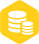# 使用51单片机读写AT29C040的C语言程序免费下载

1.1w人已加入

### 描述

＃include 《reg51.h》

＃include 《intrins.h》

unsigned char time;

void int_t0（void） interrupt 1 {

time++;

}

unsigned char d; //rec data

bit rec（void） {

TH0=0;time=0;

while （！RI） {if （time》1） return（1）;}

RI=0;

d=SBUF;

return（0）;

}

void sen（unsigned char dd） {

SBUF=dd;

while （！TI） {}

TI=0;

}

void wait（void） {

time=0;while （time《10） {}

}

void wait10ms（void） {

TH0=150;time=0;

while （！time） {}

}

sbit ce=P3^5;

sbit oe=P3^6;

sbit we=P3^7;

sbit a16=P3^2;

sbit a17=P3^3;

sbit a18=P3^4;

void pro_to_card（void） {

unsigned char no，i，j;

sen（0xaa）;

if （rec（）） {wait（）;return;}

no=d;

sen（d）;

no《《=2;

no|=0xe3;

P3=0xff;

P3&=no;

i=0;

do {

P0=i;

j=0;

do {

if （rec（）） {wait（）;ce=1;return;}

TI=0;SBUF=d;

P1=j;

P2=d;

ce=0;

we=0;

we=1;

ce=1;

j++;

} while （j）;

wait10ms（）;

i++;

} while （i）;

ce=1;

}

void card_to_pro（void） {

unsigned char no，i，j;

sen（0x55）;

if （rec（）） {wait（）;return;}

no=d;

sen（d）;

no《《=2;

no|=0xe3;

P3=0xff;

P3&=no;

P2=0xff;

i=0;

do {

P0=i;

j=0;

do {

P1=j;

ce=0;oe=0;

no=P2;

oe=1;ce=1;

j++;

sen（no）;

if （rec（）） {wait（）;return;}

if （no！=d） {wait（）;return;}

} while （j）;

i++;

} while （i）;

}

/*

void load（unsigned int as，unsigned char dd） {

P0=as/256;P1=as;P2=dd;

ce=0;we=0;we=1;ce=1;

}

void erase（void） {

a16=a17=a18=0;

wait10ms（）;

wait10ms（）;

}

void unlock（void） {

unsigned char i;

a16=a17=a18=0;

i=0;

do {

P1=i;

ce=0;we=0;we=1;ce=1;

i++;

} while （i）;

}

*/

//AT29C040读写主程序开始

void main（void） {

SCON=0x80;

TMOD=0x21;

ET0=TR0=1;EA=1;

wait10ms（）;

// erase（）;

// unlock（）;

REN=1;

while （1） {

REN=1;RI=0;

while （！RI） {} RI=0;

switch （SBUF） {

case 0x55:card_to_pro（）;break;

case 0xaa:pro_to_card（）;break;

case 0xa5：

_nop_（）;

_nop_（）;

_nop_（）;

_nop_（）;

_nop_（）;

_nop_（）;

_nop_（）;

_nop_（）;

_nop_（）;

_nop_（）;

_nop_（）;

_nop_（）;

sen（0xaa）;

break;

}

}×
20